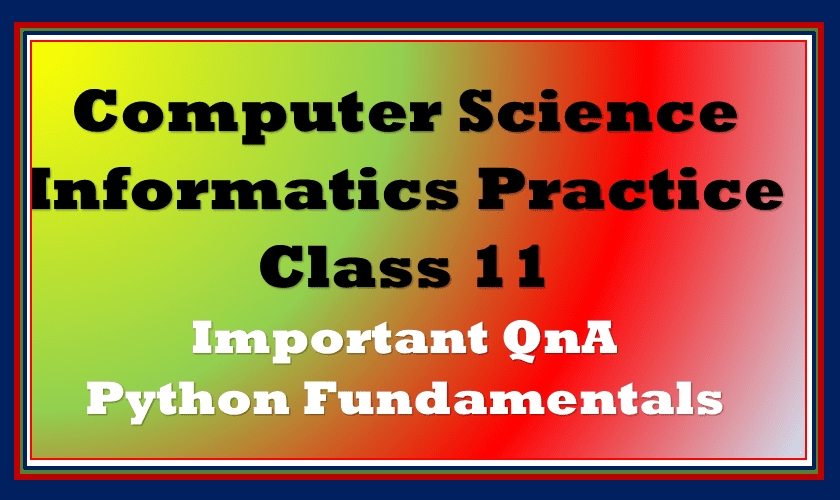In this post, we will discuss Important Python Fundamentals questions class 11 Computer Science/Informatics Practices or Introduction to python chapter. Questions based on the python fundamentals, installation of python, and the structure of the python program.

Topics Covered

## Important Python Fundamentals questions class 11

So here we start the article with Python Fundamentals questions class 11.

Q – 1 What is python?

Ans. : Python is a popular middle-level programming language but not a high-level programming language like C, C++, Java.

Q – 2 Why python is a popular programming language?

Ans.: There few reasons why python is a popular programming language, few reasons are as follows:

1. Its easy structure
2. The liveliness of code and productivity
3. No brackets in code
4. Fewer lines in code
5. Interpreted Language

Q – 3 Who developed python?

Ans.: Python was developed by Guido Van Rossum in February 1991 and then Python Software Foundation.

Q – 4 Enlist various distribution of python programming language.

Ans. The few distributions of Python programming are as follows:

1. CPython
2. PyCharm
3. Anaconda

Q – 6 How to get python and install it?

Ans. Python can be downloaded from www.python.org. Find the OS-relevant version for the computer and install it.

The next section of Python Fundamentals for class 11 provides you the questions related to IDLE.

Q – 7 What is Python IDLE?

Ans.: Python IDLE is a tool which allows writing python programs and provides the output on screen after running the program. IDLE stands for Integrated Development and Learning Environment.

Q – 8 What are the two basic modes available for writing a program in Python IDLE?

Ans.

1. Interactive Mode: Write a few lines of code and display the output
2. Script Mode: Write large programs and allows to save the program for future use

Q – 9 Write steps to how to work with interactive mode?

Ans.:

Q – 10 Write steps to work with script mode.

Ans.:

The next section of Python Fundamentals for class 11 talks about the basics of python programing.

Q – 11 What is token?

Ans.: The smallest units of a program are known as a token.

Q – 12 Which token are used in python?

Ans.: The tokens used in python are as following:

1. Keywords
2. Literals
3. Identifiers
4. Punctuators
5. Operator

Q – 13 How to write multiple lines code in python? or What are the ways to write multiple lines code in python?

Ans.: Python allows two ways of writing code in multiple lines in a program:

By using double quotes and slash

``````>>> str = "This is an example of multiple line \
Code in \
Python"``````

By using triple double quotes

``````>>> str = """This is an example of multiple line
of code in
Python"""``````

In the next section of Python Fundamentals for class 11 is all about data types in python.

Q – 14 What is the use of None in Python?

Ans.: None is a special literal used in python. It means there is no value stored in an identifier. In other words, None means the that value is not assigned to the identifier.

Q – 15 Identify the types of the identifier of the following: 15.859, 125, True, ‘True’, False, ‘False’, 0XDADA, 0o456, None, “None”

Ans. :

Q – 16 Differentiate between an expression and a statement.

Ans.:

The next section of Python Fundamentals for class 11 talks about error finding questions.

Q – 17 Find out errors in the following

1

``````x = 090
y = 0o123
z = 0o99
print x
print(y)
print (x AND y AND z)
``````

Ans.:

Errors:

Line 1: The variable x should initialize a number starting with 0 because python doesn’t allow to do the same.

Line 3: When a value starts with 0o it represents the octal value and octal value is in the range of 0 to 8. In this line, z is initialized with 99 which is out of range.

Line 5: print() function required comma-separated value to print.

2

``````p,q,r= 33, 44, 66
print(p;q;r)``````

Ans.: Same as above Line 5 explanation.

3

``````n=input("Enter no.")
sq_of_n=n*n
print(sq_of_n)
``````

Ans.:

Runtime error inline 1 as input() function returns only string that doesn’t allow computation. To allow computation int the statement use int(), the correct would be like this:

n=int(input(“Enter no.:”))

4

``````cname=int("Enter Name:")
bill=int(input("Enter amoumnt:"))
print(cname)
print(bill)
``````

Ans.:

In input() function is required in place of int. Line 3 and line 4 should be used with the same indent level as other lines.

Correct Code:

``````cname=input("Enter Name:")
bill=int(input("Enter amoumnt:"))
print(cname)
print(bill)``````

## FAQs on Python Fundamentals questions class 11

### Is Python taught in class 11?

Yes, python taught in class 11 for CBSE Computer Science and Informatics practices curriculum. Moreover if student has opted Artificial Intelligence in Class 9 and 10 Python fundamentals are there to learn.

### What is Python for class 11th?

Python is a programming language taught in CBSE Computer Science and Informatics Practices Syllabus.

### Which book is best for Python Class 11?

There few very popular books available in the market. Some of them are:
1. NCERT Textbook for Computer Science Class 11
2. NCERT Textbook for Informatics Practices Class 11
3. Computer Science Textbook for Class 11 – Sumita Arora
4. Computer Science Textbook for Class 11 – Preeti Arora

### What are the basic fundamentals of Python?

Basic fundamentals of Python refers to the basics of programming. Here students will learn the fundamental concepts of python program. They learn how to write program and execute the program in python environment.